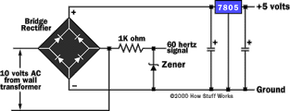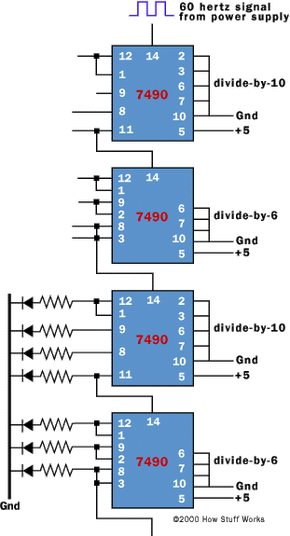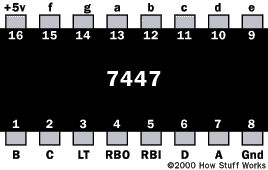# How Digital Clocks Work

Chances are that in your bedroom you have a digital clock beside your bed. Have you ever looked at it in the morning and wondered how it works?

In this article, you're going to learn exactly how a digital clock (or wristwatch) works. In fact, you're even going to learn how to build your own!

To understand how a digital clock works, you have to get inside and see what's going on. So let's get started!

## The Basics

If you have read How Pendulum Clocks Work, you know that all clocks (regardless of technology) have a few required components:

• A source of power to run the clock In a pendulum clock, the weights or the springs handle this role.
• An accurate timebase that acts as the clock's heartbeat In a pendulum clock, the pendulum and escapement handle this role.
• A way to gear down the timebase to extract different components of time (hours, minutes, seconds) In a pendulum clock, gears serve this role.
• A way to display the time In a pendulum clock, the hands and face serve this role.

A digital clock is no different. It simply handles these functions electronically rather than mechanically. So in a digital clock, there is an electrical power supply (either a battery or 120-volt AC power from the wall). There is an electronic timebase that "ticks" at some known and accurate rate. There is an electronic "gearing mechanism" of some sort -- generally a digital clock handles gearing with a component called a "counter." And there is a display, usually either LEDs (light emitting diodes) or an LCD (liquid crystal display).

## High-Level View

Here is a quick overview of the components of a digital clock at a high level.

At the heart of the clock there is a piece that can generate an accurate 60-hertz (Hz, oscillations per second) signal. There are two ways to generate this signal:

1. The signal can be extracted from the 60-Hz oscillations in a normal power line. Many clocks that get their power from a wall socket use this technique because it is cheap and easy. The 60-Hz signal on the power line is reasonably accurate for this purpose.
2. The signal can be generated using a crystal oscillator. Obviously, any battery-operated clock or wristwatch will use this technique instead. It takes more parts, but is generally much more accurate.

The 60-Hz signal is divided down using a counter. When building your own clock, a typical TTL part to use is a 7490 decade counter. This part can be configured to divide by any number between 2 and 10, and generates a binary number as output. So you take your 60-Hz time base, divide it by 10, divide it by 6 and now you have a 1-Hz (1 oscillation per second) signal. This 1-Hz signal is perfect for driving the "second hand" portion of the display. So far, the clock looks like this in a block diagram:To actually see the seconds, then the output of the counters needs to drive a display. The two counters produce binary numbers. The divide-by-10 counter is producing a 0-1-2-3-4-5-6-7-8-9 sequence on its outputs, while the divide-by-6 counter is producing a 0-1-2-3-4-5 sequence on its outputs. We want to display these binary numbers on something called a 7-segment display. A 7-segment display has seven bars on it, and by turning on different bars you can display different numbers:To convert a binary number between 0 and 9 to the appropriate signals to drive a 7-segment display, you use a (appropriately named) "binary number to 7-segment display converter." This chip looks at the binary number coming in and turns on the appropriate bars in the 7-segment LED to display that number. If we are displaying the seconds, then the seconds part of our clock looks like this:The output from this stage oscillates at a frequency of one-cycle-per-minute. You can imagine that the minutes section of the clock looks exactly the same. Finally, the hours section looks almost the same except that the divide-by-6 counter is replaced by a divide-by-2 counter.

Now there are two details left to figure out if you are building a real clock:

• The clock as designed here does not understand that at 12:59:59 it is supposed to cycle back to 1:00. That is a messy little problem, and there are a couple of ways to solve it. One technique involves creating a little bit of logic that can detect the number 13 and reset the hour section back to 1 (not zero). Another technique involves using an adder. For our purposes, it is easier to deal in military time, because military time includes a zero hour.
• We need a way to set the clock. Typically this is handled by gating higher-than-normal frequencies into the minutes section. For example, most clocks have "fast" and "slow" set buttons. When you press the "fast" button, the 60-Hz signal is driven straight into the minutes counter. When you press the "slow" button, a 1-Hz signal is driven into the minutes section. There are other possible techniques, but this one is the most common.

Now let's see what we have to do to build a real clock!

## Building Your Own Digital Clock

The best way to understand the different components of a digital clock and how they work together is to actually walk through the steps of building your own clock. Here we will build just the "seconds" part of the clock, but you can easily extend things to build a complete clock with hours, minutes and seconds. To understand these steps, you will need to have read How Boolean Logic Works and How Electronic Gates Work. In particular, the electronic gates article introduces you to TTL chips, breadboards and power supplies. If you have already played around with gates as described in that article, then the description here will make a lot more sense.

The first thing we need is a power supply. We built one in the electronic gates article. That time, we used a standard wall transformer that produced DC (direct current) power and then regulated it to 5 volts using a 7805. For our clock, we want to do things slightly differently because we are going to extract our 60-Hz timebase from the power line. That means that we want an AC rather than a DC transformer, and we will use a part called a bridge rectifier to convert the AC to DC. Therefore, we need the following parts for our power supply:

• 12-volt AC transformer (Jameco part #115602)
• Bridge rectifier (Jameco part #103018)
• 7805 5-volt regulator (TO-220 case) (Jameco part #51262)
• Two 470-microfarad electrolytic capacitors (Jameco part #93817)
• 5.1-volt zener diode (Jameco part #36097)
• 1-K-ohm resistor (Jameco part #29663)

A few notes on the parts used:

• The difference between the AC transformer we are using here and the DC transformer we used in the article on gates is that the AC transformer preserves the 60-Hz sine wave found in 120-volt household current. If you want to use your volt-ohm meter to measure the voltage of an AC transformer, be sure you use an AC voltage range rather than a DC range.
• We use the bridge rectifier to convert the AC to DC. One of the terminals on the rectifier will be marked with a "+" -- from that you can find the minus and AC inputs. There is no polarity to an AC transformer, so it does not matter which transformer lead you connect to which AC lead of the rectifier.
• The 7805 and capacitors are wired just like they were in the electronic gates article.
• The resistor and the zener diode extract a 60-Hz signal from the transformer's sine wave. A diode is a one-way valve for electrons. A zener diode is also a one-way valve, but it also passes electrons in the other direction if they are above a certain voltage. The zener diode therefore turns a 10-volt sine wave into a clipped wave oscillating between 0 and 5 volts. This is perfect for clocking the TTL counters. The 1-K-ohm resistor makes sure that the current to the zener diode is limited so we do not burn out the diode. The diode will have a band painted on one end -- this band should be the end connected to the resistor.

## Circuit DiagramHere's a circuit diagram for the power supply and time base.

As we saw in the article on electronic gates, the power supply is the most difficult part!

To create the rest of the clock you will need:

• At least four 7490 or 74LS90 chips
• At least two 7447 or 74LS47 binary-to-7-segment converters
• At least 20 resistors for the LEDs in the 7-segment displays (330 ohms would be fine.)
• Some normal LEDs
• At least two common-anode (CA) 7-segment LED displays (Jameco part # 17208 is typical.)

The number of chips, resistors and LEDs you need depends on how many digits you are interested in implementing. Here we will discuss only seconds, so the "at least" numbers are correct.

## 7490 Pinout

Let's look at the 7490 briefly to see how it works.

The 7490 is a decade counter, meaning it is able to count from 0 to 9 cyclically, and that is its natural mode. That is, QA, QB, QC and QD are 4 bits in a binary number, and these pins cycle through 0 to 9, like this:

```QD	QC	QB	QA
0	0	0	0
0	0	0	1
0	0	1	0
0	0	1	1
0	1	0	0
0	1	0	1
0	1	1	0
0	1	1	1
1	0	0	0
1	0	0	1
```You can also set the chip up to count up to other maximum numbers and then return to zero. You "set it up" by changing the wiring of the R01, R02, R91 and R92 lines. If both R01 and R02 are 1 (5 volts) and either R91 or R92 are 0 (ground), then the chip will reset QA, QB, QC and QD to 0. If both R91 and R92 are 1 (5 volts), then the count on QA, QB, QC and QD goes to 1001 (5). So:

• To create a divide-by-10 counter, you first connect pin 5 to +5 volts and pin 10 to ground to power the chip. Then you connect pin 12 to pin 1 and ground pins 2, 3, 6, and 7. You run the input clock signal (from the timebase or a previous counter) in on pin 14. The output appears on QA, QB, QC and QD. Use the output on pin 11 to connect to the next stage.
• To create a divide-by-6 counter, you first connect pin 5 to +5 volts and pin 10 to ground to power the chip. Then you connect pin 12 to pin 1 and ground pins 6 and 7. Connect pin 2 to pin 9 and pin 3 to pin 8. Run the input clock signal (from the timebase or a previous counter) in on pin 14. The output appears on QA, QB and QC. Use pin 8 to connect to the next stage.

## Creating the Second HandKnowing all of this, you can easily create the "second hand" of a digital clock. It looks like this:

In this diagram, the top two 7490s divide the 60-Hz signal from the power supply down by a factor of 60. The third 7490 takes a 1-hertz signal as input and divides it by 10. Its four outputs drive normal LEDs in this diagram. The fourth 7490 divides the output of the third by 6, and its three outputs drive normal LEDs as well. What you have at this point is a "second hand" for your clock, with the output of the second hand appearing in binary. If you would like to create a clock that displays the time in binary, then you are set! Here is a view of a breadboard containing a divide-by-10 counter, a divide-by-6 counter and a set of LEDs to display the output of the counters in binary:The wires entering the picture from the left are power, ground and clock lines for the board. The left counter is a 7490 set up to divide by 10, and the right one is another 7490 set up to divide by 6.

If you pack things tighter and do a neater wiring job, you can fit up to four counters on a single breadboard.

## Displaying the Time as NumeralsIf you want to display the time as numerals, you need to use the 7447s. Here is the pinout of a 7447, as well as the segment labeling for a 7-segment LED.You connect a 7447 to a 7490 like this:

• Provide +5 volts on pin 16 and ground on pin 8 to power the 7447 chip.
• Connect QA, QB, QC and QD from a 7490 to pins 7, 1, 2 and 6 of the 7447, respectively.
• Connect 330-ohm resistors to pins 13, 12, 11, 10, 9, 15 and 14 of the 7447, and connect those resistors to the a, b, c, d, e, f, and g segments of the 7-segment LED.
• Connect the common anode of the 7-segment LED to +5 volts.You will need to have the pinout for the specific LED display that you use so that you know how to wire the outputs of the 7447 to the LEDs in the 7-segment device. (Also, note that the 7448 is equivalent to the 7447 except that it drives common-cathode displays. Ground the common cathode of the LED in that case.)

You can see that by extending the circuit, we can easily create a complete clock. To create the "minute hand" section of the clock, all that you need to do is duplicate the "second hand" portion. To create the "hour hand" portion, you are going to want to be creative. Probably the easiest solution is to create a clock that displays military time. Then you will want to use an AND gate (or the R inputs of the 7490) to recognize the binary number 24 and use the output of the recognizer to reset the hour counters to zero.

The final piece you need to create is a setting mechanism. On a breadboard, it is easy to set the clock -- just move the input wires to drive higher-frequency signals into the minute-hand section of the clock. In a real clock, you would use pushbuttons or switches and gates to do the same thing.

If you happen to take your bedside clock or watch apart, one thing you will notice is that there are probably not 15 TTL ICs inside. In fact, you may not be able to find a chip at all. In most modern clocks and watches, all of the functions of the clock (including the alarm and any other features) are all integrated into one low-power chip (in a watch, the chip and display together consume only about a millionth of a watt). That chip is probably embedded directly into the circuit board. You might be able to see a blob of black plastic protecting this chip. That one tiny chip contains all of the components we have discussed here.

Now you have a complete understanding of how digital clocks work. The next time you look at the clock beside your bed or at your digital wristwatch, you can do so with a new respect for what is going on inside! If you would like to advance to the next level and see how to build a digital clock with a microcontroller, see How Microcontrollers Work.